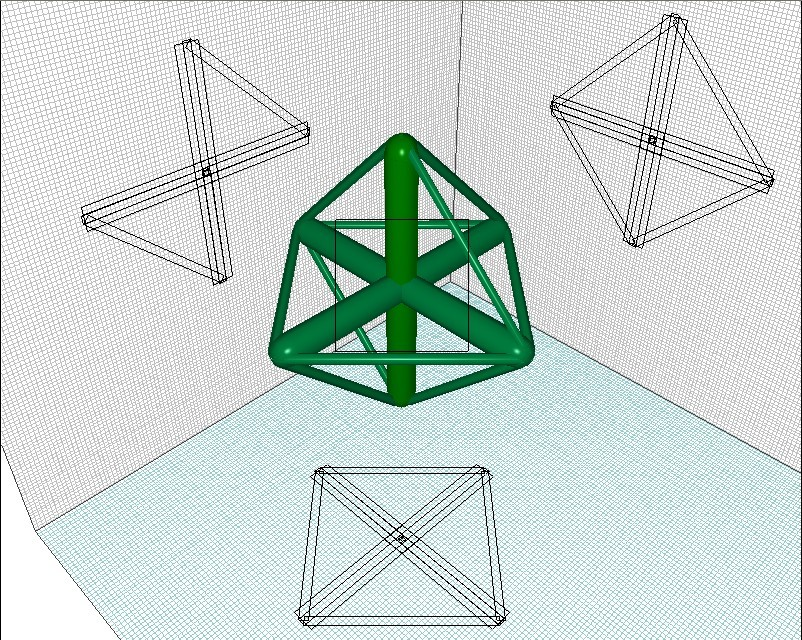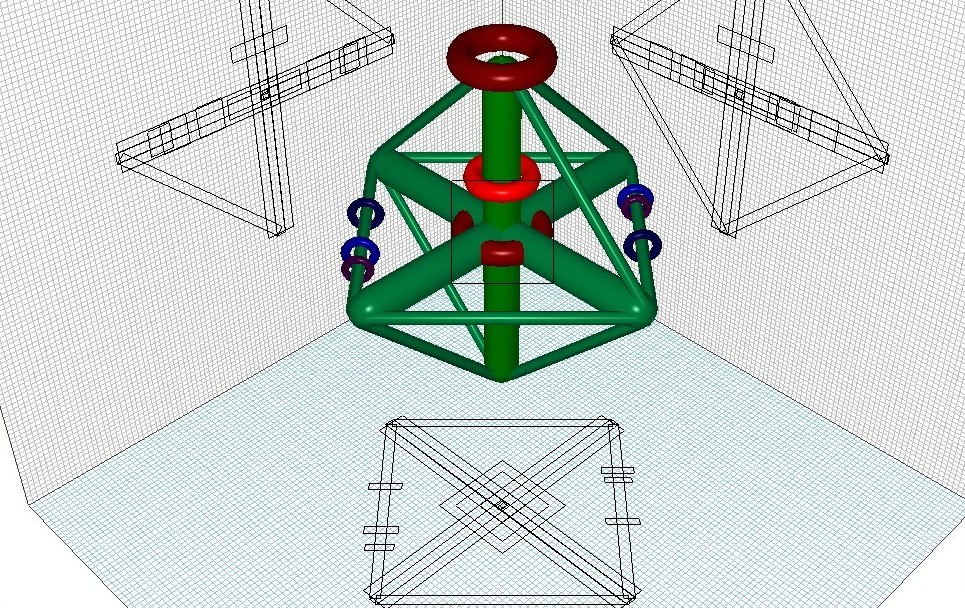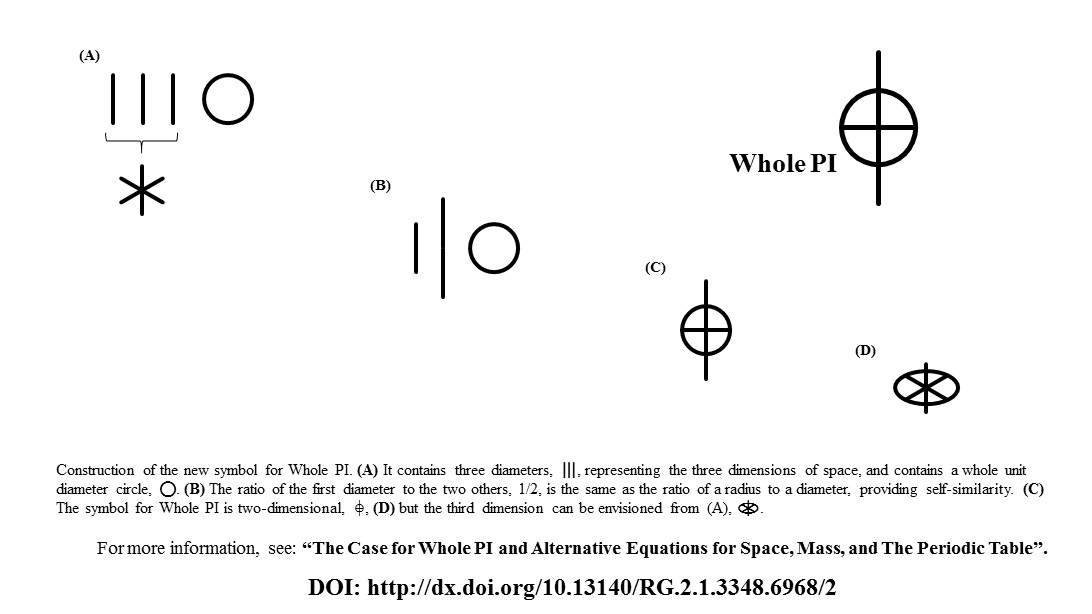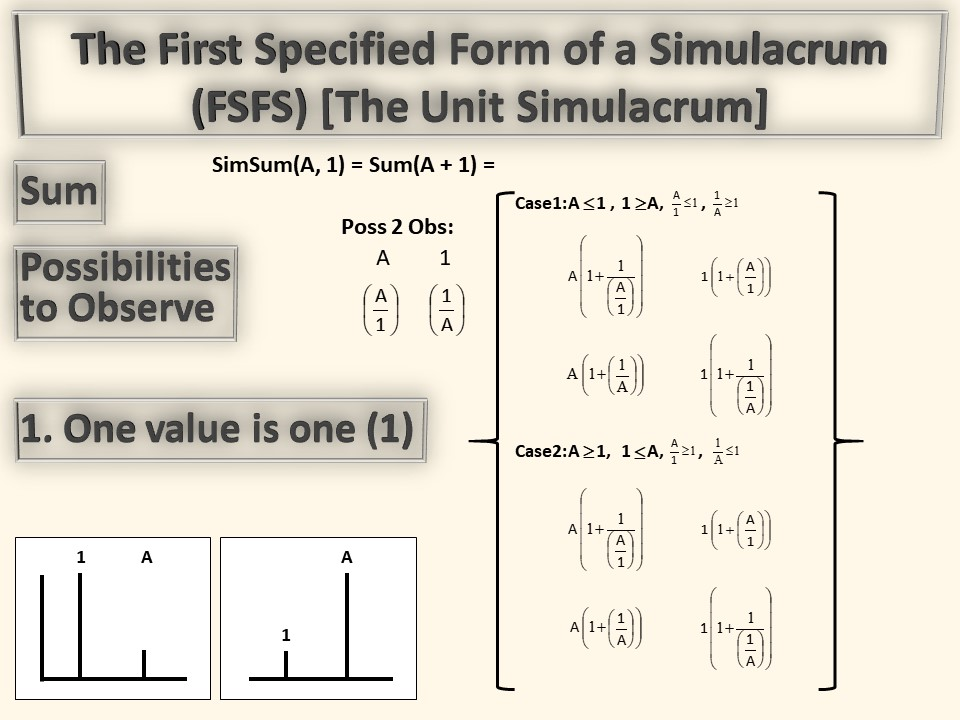# The Unit Simulacrum is the First Specificed Form of a Simulacrum

The First Specified Value is 1. One of the two variables is set to the value of 1.

## A Simulacrum Sum is the sum of any two variables, values, ratios, words, text, icons, emojis, or any other physical representations

### The Simulacrum System

The Simulacrum System has several inherent constructs known as Critical Ratios.

The Simulacrum System consists of:

Fulll General Form of a Simulacrum (FuGFS)
First General Form of a Simulacrum (FGFS)
Anti-General Form of a Simulacrum (AGFS)

The Full General Form of a Simulacrum is The Matrix

The First General Form of a Simulacrum (FGFS) is The Simulacrum

The Anti-General Form of a Simulacrum (AGFS) is The Anti Simulacrum

The First General Form of a Simulacrum (FGFS), The Simulacrum, is where all of "normal Reality" exists. Everything is a Simulacrum.
Normal Reality plus the ParaNormal is The Matrix, and can be defined as The Simulacrum plus the The Anti Simulacrum. The RIght SIde Up and The Upside Down.

Normal Reality is defined by the Interpretation Matrix
The Interpretation Matrix is the list of rules for the symbols used to form The Construct, or to construct The Simulacrum. Each symbol for Reality has a Unit Definition, The First One.

We start with an inherent package of installed definitions, and develop or adopt others, including: Human, Conscious, on Earth, English language, alphabet, numerical system, knowledge of words, education, etc.
Everything is relative to the definitions of the time in which they were defined.
2004 was The First Time The Simulacrum System became a New Thoughtform. That was  The First Simulacrum System Time

Simulacrum System Time

Simulacrum System Time started in 2004, with the discovery by Dr. Byrdwell of The Bottom Up Solution, The Simulacrum System, and Whole PI.

The First Step, The First Deconstruction, was The Bottom Up Solution, discovered in 2004, and published in 2005, as The Bottom Up Solution to the Triacylglycerol Lipidome [link goes to AOCS (American Oil Chemists' Society) Lipids journal page for the Bottom Up Solution].
That was the First Official Report of The First Deconstruction of The Simulacrum.
The Bottom Up Solution was The First Deconstruction of The Triacylglycerol Simulacrum System reported.

I discovered the Deconstruction first. I then generalized it to see The Simulacrum.
The Bottom Up Solution was named such because The Simulacrum had been discovered.The Bottom Up SolutionThe Bottom Up Solution to the Triacylglycerol Lipidome

The Simulacrum was discovered in 2004, soon after the Bottom Up Solution.
But The Simulacrum System was not published until later. "The Simulacrum System as a Construct for Mass Spectrometry of Triacylglycerols and Others" was published in 2016, also in the journal Lipids;The Simulacrum

In between the Bottom Up Solution and The Simulacrum System were two examples of The Updated Bottom Up Solution, one using APCI-MS and one using APPI-MS & ESI-MS. See the bottom of this page for those references.

After discovering The Simulacrum and seeing the Fibonacci Ratios in the Triacylglycerol Lipidome, I started looking at other transcendental ratios. Pi, π, is a transcendental number that has always intrigued man. So I constructed The Pi Simulacrum. This led to The Pi Paradox and Whole PI. See the WholePI page.

Whole PI has not yet been published in a peer-reviewed mathematics, chemistry, physics, or other journal. No reviewer has ever proven any error in The Case for Whole PI, but they just haven't published it (in a journal I consider reputable).

"The Case for Whole PI and Alternative Equations for Space, Mass, and The Periodic Table" is available online in multiple places:

1. Researchgate.net: https://www.researchgate.net/profile/William-Byrdwell
2. Directly from Byrdwell.com: https://www.Byrdwell.com/publications.html
3. And as "A Simple Model with a Unified Form of Equations for Space, Mass, and the Periodic Table" at Vixra.org (the opposite of Arxiv.org), at: https://vixra.org/abs/1907.0172Whole PI

Back to The SimulacrumCritical Ratios and Critical Limits

The First General Form of a Simulacrum (FGFS) has Critical Ratios that define Critical Limits. Several defining simulacra constitute Critical Limits.
Critical Limits are Special Cases, as follows:
First General Form of a Simulacrum (FGFS) has Special Cases:
Null Null Simulacrum = SimSum(0,0) = SimSum(0,0)
Null Unit Simulacrum = Unit Null Simulacrum = SimSum(0,1) = SimSum(1,0)
Unit Unit Simulacrum = SimSum(1,1) = SimSum(1,1)
Unit Anything Simulacrum = SimSum(1,Anything) = SimSum(Anything,1)
Unit Ratio Simulacrum = SimSum(1,Ratio) = SimSum(Ratio,1)
Unit Everything Simulacrum = SimSum(1,Everything) = SimSum(Everything,1)
Anything anything Simulacrum = SimSum(Anything,anything) = SimSum(anything,Anything)
Ratio ratio Simulacrum = SimSum(Ratio,ratio) = SimSum(ratio,Ratio)
Everything everything Simulacrum = SimSum(Everything,everything) = SimSum(everything,Everything)

The Anti-General Form of a Simulacrum (AGFS) is The Anti Simulacrum
The Anti-General Form of a Simulacrum (AGFS) contains the same Special Cases as the First Generail Form of a Simulacrum (FGFS)

The First General Form of a Simulacrum (FGFS), or simply
The Simulacrum is:

The Simulacrum Sum of A and B = SimSum(A,B) = Sum ( A + B ) =
{Possibilities to Observe: A, B, (A/B), (B/A);
Case 1: A <= B, B >= A, (A/B) <= 1, (B/A) >= 1;
A ( 1 + 1/(A/B) )  or  B ( 1 + (A/B) )
A ( 1 + (B/A) )  or  B ( 1 + 1/(B/A) )
Case 2: A >= B, B <= A, (A/B) >= 1, (B/A) <= 1;
A ( 1 + 1/(A/B) ) or B ( 1 + (A/B) )
A ( 1 + (B/A) ) or B ( 1 + 1/(B/A) ) }

Interpretation Matrix: Definitions of A and B and relationships between them. A and B are ratios to their unit definitions. You can construct the ratios of the variables, A and B, which are ratios to their unit definitions. Thus, The Simulacrum is Ratios of Ratios.The Full General Form of a Simulacrum (FuGFS) is The First General Form of a Simulacrum (FGFS) plus The Anti-General Form of a Simulacrum (AGFS)

The First General Form of a Simulacrum is half of the Full General Form of a Simulacrum. The Full General Form of a Simulacrum (FuGFS) consists of the First General Form of a Simulacrum (FGFS) and the Anti General Form of a Simulacrum (AGFS). The Anti General Form of a Simulacrum has the opposite definitions of Cases, namely Case 1: B <= A, A >= B, (A/B) >= 1, (B/A) <= 1, etc.
All of "normal reality" fits within the First Genreal Form of a Simulacrum; that's why it's simply called The Simulacrum.
For instance, in The Simulacrum, (1/2) <= 1 is Case 1. In the Anti Simulacrum, (1/2) >= 1 is Case 1. The Anti-Simulacrum exists to account for all possibilities, whether real or unreal, observed or unobserved, correctly defined or incorrectly defined, Obverse and Inverse perspectives.

Since "Normal Reality" has 1/2 <= 1 as Case 1, The Simulacrum is said to be 'Aligned', having a lesser value be the lesser Case.
The Anti Simulacrum is UnAligned.

The Octagon is the symbol for The Simulacrum because the eight sides represent the eight Solutions in the two Cases of The Simulacrum. The lower and upper halves of The Simulacrum represent Case 1 and Case 2, respectively.
The lower four edges are Case 1, A < B, (A/B) < 1
The upper four edges are Case 2, A > B, (A/B) > 1
The equatorial four edges are Case 1 = Case 2, A = B, (A/B) = (B/A) = 1

### The Unit Simulacrum

If you make one specification to the First General Form of a Simulacrum, the first specification is that one value equals one. If you specify that one value equals 1, you get the First Specified Form of a Simulacrum. Since one value is a unit (1), the First Specified Form of a Simulacrum is known as The Unit Simulacrum.

The First Specified Form of a Simulacrum (FSFS),
or simply
The Unit Simulacrum is:

The Simulacrum Sum of 1 and A = SimSum(1,A) = Sum ( 1 + A ) =
{Possibilities to Observe: 1, A, (1/A), (A/1);
Case 1: A <= 1, 1 >= A, (A/1) <= 1, (1/A) >= 1;
A ( 1 + 1/(A/1) ) or 1 ( 1 + (A/1) )
A ( 1 + (1/A) ) or 1 ( 1 + 1/(1/A) )
Case 2: A >= 1, 1 <= A, (A/1) >= 1, (1/A) <= 1;
A ( 1 + 1/(A/1) ) or 1 ( 1 + (A/1) )
A ( 1 + (1/A) ) or 1 ( 1 + 1/(1/A) ) }

Interpretation Matrix: Definitions of 1 and A and relationships between them. 1 can be defined as the unit that defines A, making 1+ A The Unit Definition of A, also known as The Unit Simulacrum of A.

Since 1 + A = A + 1, The Unit Simulacrum is also known as The First Increment.
When you add 1 to something you increment that something.

1 + 1 is the First Increment of 1
1/1 = 1
1/1 + 1 is the First Increment of 1/1
1/(1+1) = 1/2 is the First Decrement of 1/1

Again, the Octagon is the symbol for The Simulacrum because the eight sides represent the eight Solutions in the two Cases of The Simulacrum. The lower and upper halves of The Simulacrum represent Case 1 and Case 2, respectively.One shortcut used for The Unit Simulacrum is that we always select the easiest solutions. We choose the solutions that have the '1' outside the parentheses, because the '1' cancels out and disappears, allowing the parentheses to be discarded. These are called the Simplified Solutions.

The Simplified Solutions end up being
1 + (ratio/1) and 1 + 1/(1/ratio)

Thus, out of eight possible solutions, we end up with two solutions, giving two answers based on whether the ratio is observed directly or indirectly (1/ratio).

The simulacrum is a looking Glass to examine everything in a new way.
Construct "Critical Ratios" to Extract information from data.

### Publications

The Simulacrum System was discovered step-by-step, from analysis of fats and oils using high-tech instruments and techniques (liquid chromatography and mass spectrometry). I constructed Critical Ratios that provided structural information about triglycerides (triacylglycerols) and how they're metabolized by the human body.

The first step was The Bottom Up Solution.
Then The Updated Bottom Up Solution.
Then The Simulacrum Sytem.
Then Whole PI.

1. Byrdwell, W.C., "The Bottom Up Solution to the Triacylglycerol Lipidome Using Atmospheric Pressure Chemical Ionization Mass Spectrometry", Lipids, 40(4):383-417 (2005), DOI: 10.1007/s11745-006-1398-9.
2. Byrdwell, W. C., "The Updated Bottom Up Solution Applied to Mass Spectrometry of Soybean Oil in a Dietary Supplement Gelcap", Analytical and Bioanalytical Chemistry, 407(17):5143-5160 (2015), DOI: 10.1007/s00216-015-8590-9.
3. Byrdwell, W. C., "The Updated Bottom Up Solution Applied to Atmospheric Pressure Photoionization and Electrospray Ionization Mass Spectrometry", Journal of the American Oil Chemists' Society, 92(11):1533-1547 (2015), DOI: 10.1007/s11746-015-2735-z.
4. Byrdwell, W. C., "The Simulacrum System as a Construct for Mass Spectrometry of Triacylglycerols and Others", Lipids, 51(2):211-227 (2016), DOI: 10.1007/s11745-015-4101-1.
5. Byrdwell, W. C., "The Case for Whole PI and Alternative Equations for Space, Mass, and the Periodic Table", ResearchGate, DOI: 10.13140/RG.2.1.3348.6968/2.

These and other publications by Dr. Wm. Craig Byrdwell are available at: www.Byrdwell.com/Publications.html